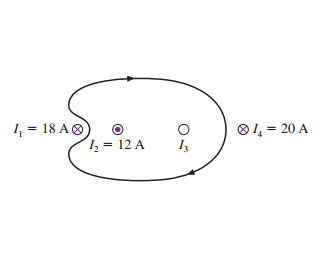# Problem: The value of the line integral around the closed path in the figure is 1.70×10−5 Tm .a. What is the direction (in or out of the page) of I3?b. What is the magnitude of I3?

###### FREE Expert Solution

Ampere's law:

$\overline{){\mathbf{\oint }}{\mathbf{B}}{\mathbf{·}}{\mathbf{d}}{\mathbf{s}}{\mathbf{=}}{{\mathbf{\mu }}}_{{\mathbf{0}}}{{\mathbf{I}}}_{\mathbf{e}\mathbf{n}\mathbf{c}\mathbf{l}\mathbf{o}\mathbf{s}\mathbf{e}\mathbf{d}}}$, where permeability, μ0 = 4π × 10-7 H/m

(a)

We use the right-hand rule to determine the direction of the current, I3.

The magnetic field is moving in a clockwise direction around the loop.###### Problem DetailsThe value of the line integral around the closed path in the figure is 1.70×10−5 Tm .

a. What is the direction (in or out of the page) of I3?

b. What is the magnitude of I3?

Frequently Asked Questions

What scientific concept do you need to know in order to solve this problem?

Our tutors have indicated that to solve this problem you will need to apply the Ampere's Law (Calculus) concept. You can view video lessons to learn Ampere's Law (Calculus). Or if you need more Ampere's Law (Calculus) practice, you can also practice Ampere's Law (Calculus) practice problems.

What professor is this problem relevant for?

Based on our data, we think this problem is relevant for Professor Shoemaker's class at TEXAS.